Ex 10.1

Chapter 10 Class 12 Vector Algebra
Serial order wise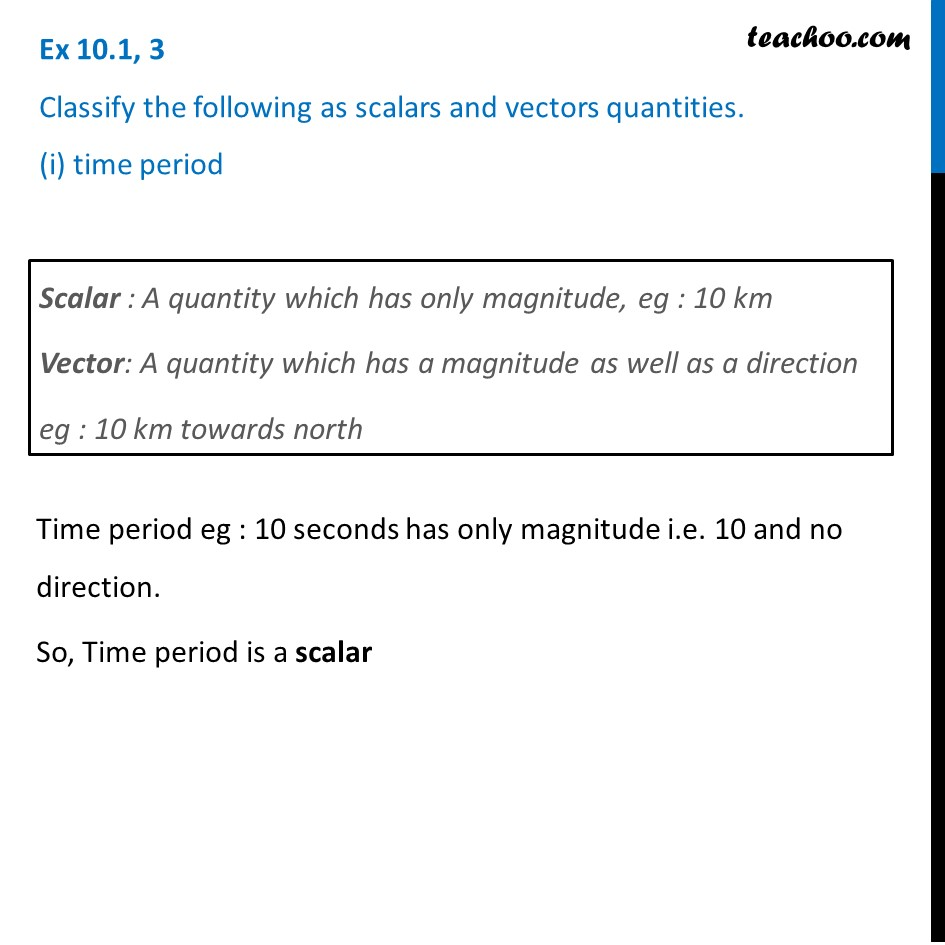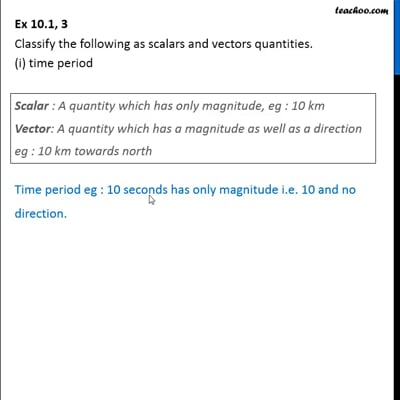This video is only available for Teachoo black users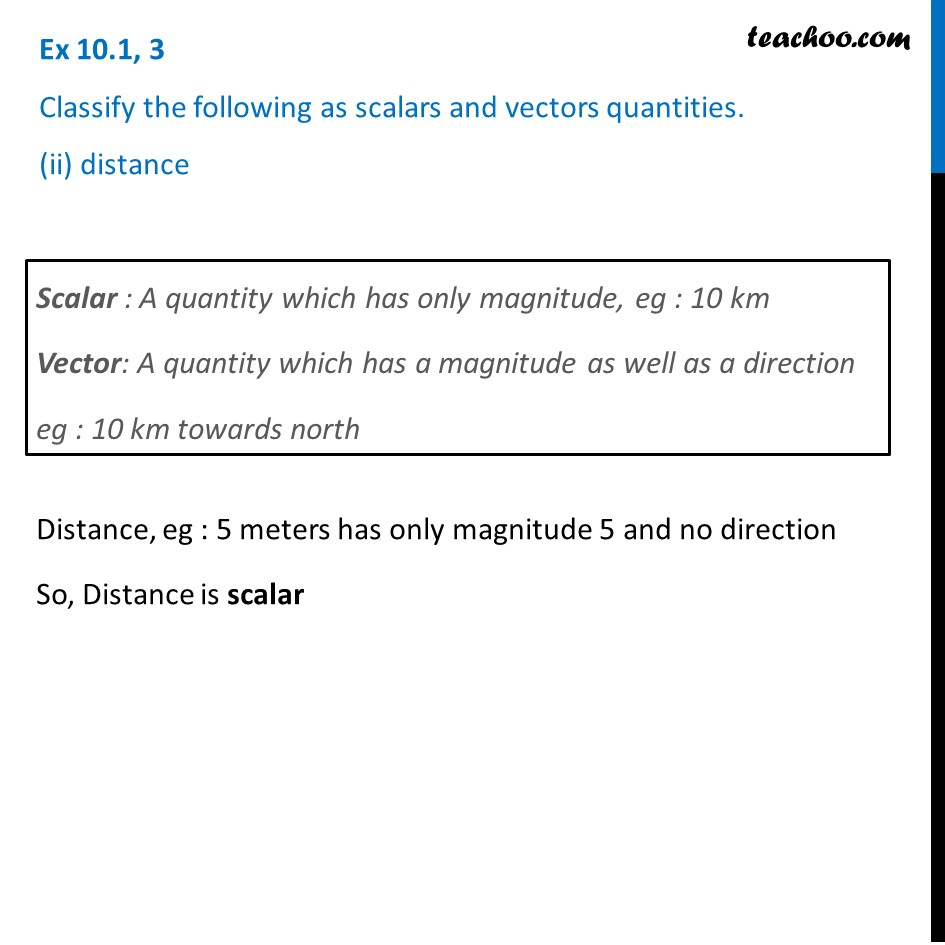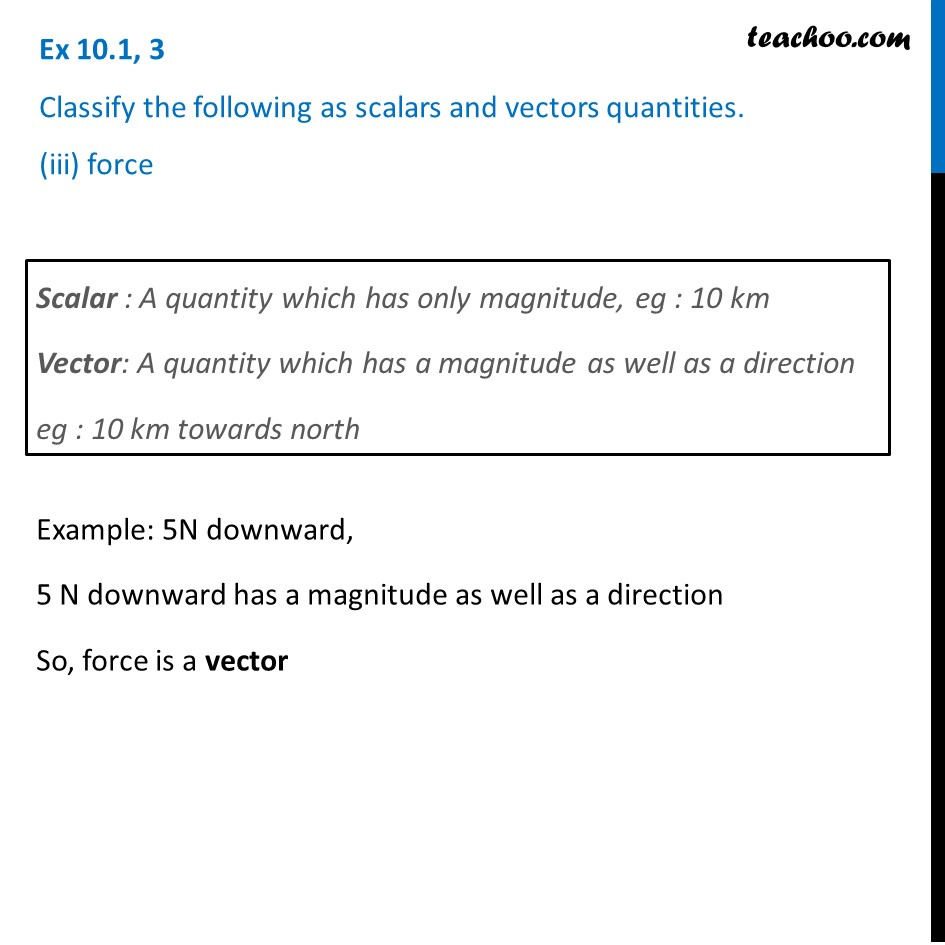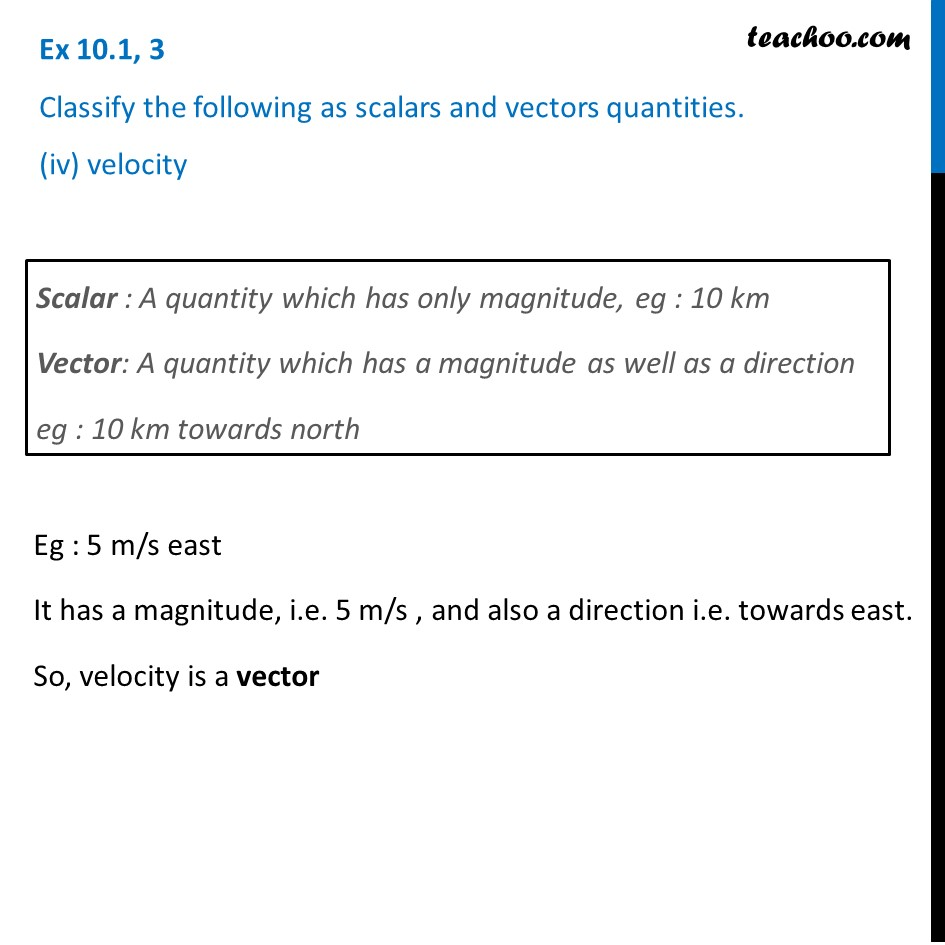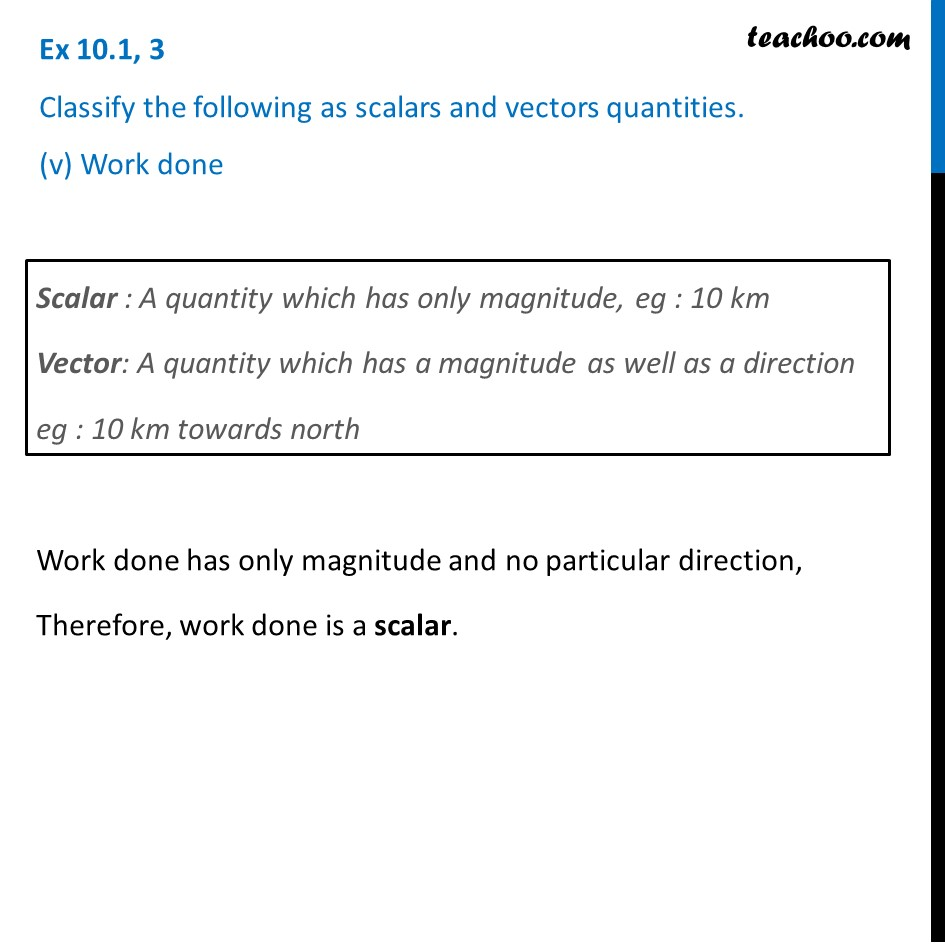Introducing your new favourite teacher - Teachoo Black, at only ₹83 per month

### Transcript

Ex 10.1, 3 Classify the following as scalars and vectors quantities. (i) time periodScalar : A quantity which has only magnitude, eg : 10 km Vector: A quantity which has a magnitude as well as a direction eg : 10 km towards north Time period eg : 10 seconds has only magnitude i.e. 10 and no direction. So, Time period is a scalar Ex 10.1, 3 Classify the following as scalars and vectors quantities. (ii) distanceScalar : A quantity which has only magnitude, eg : 10 km Vector: A quantity which has a magnitude as well as a direction eg : 10 km towards north Distance, eg : 5 meters has only magnitude 5 and no direction So, Distance is scalar Ex 10.1, 3 Classify the following as scalars and vectors quantities. (iii) forceScalar : A quantity which has only magnitude, eg : 10 km Vector: A quantity which has a magnitude as well as a direction eg : 10 km towards north Example: 5N downward, 5 N downward has a magnitude as well as a direction So, force is a vector Ex 10.1, 3 Classify the following as scalars and vectors quantities. (iv) velocityScalar : A quantity which has only magnitude, eg : 10 km Vector: A quantity which has a magnitude as well as a direction eg : 10 km towards north Eg : 5 m/s east It has a magnitude, i.e. 5 m/s , and also a direction i.e. towards east. So, velocity is a vector Ex 10.1, 3 Classify the following as scalars and vectors quantities. (v) Work doneScalar : A quantity which has only magnitude, eg : 10 km Vector: A quantity which has a magnitude as well as a direction eg : 10 km towards north Work done has only magnitude and no particular direction, Therefore, work done is a scalar.